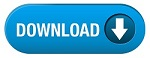Home » SSC » Model Questions for Scientific Assistant (IMD) Exam (Paper-2) Physics – Set 17

# Model Questions for Scientific Assistant (IMD) Exam (Paper-2) Physics – Set 17

## 1. The stretching of an elastic material is proportional to the force that is applied.

a. Atomic bonding
b. Density
c. Elasticity
d. Hooke’s law

## 2. An object changes shape when a force is applied to it, but when the force is removed, the deformation disappears.

a. Atomic bonding
b. Density
c. Elasticity
d. Hooke’s law

## 3. Study of how relationships among weight, surface, strength are affected by changing size of an object.

a. Atomic bonding
b. Density
c. Elasticity
d. Scaling

## 4. Which has more surface area?

a. a whole potato
b. a potato cut into four quarters
c. a potato cut into Freedom fries (ex-French fries)
d. all of these have the same surface area

## 5. Doubling the length of the sides of a cube multiplies its volume by

a. 2 and its surface area by 1
b. 4 and its surface area by 2
c. 6 and its surface area by 3
d. 8 and its surface area by 4

#a. 1
b. 1/2
c. 1/4
d. 1/8

## 7. Which has greater surface area for a given body weight (i.e., greater surface area per unit weight)

a. a snake
b. an elephant
c. a bowling ball
d. a piece of string

## 8. An object has a mass of 60 kg and a density of 30 kg per cubic meter. What is the volume of the object?

a. 1800 cubic meters
b. 2 cubic meters
c. 30 cubic meters
d. 60 cubic meters

## 9. Which has more surface area?

a. a whole potato
b. a potato cut into four quarters
c. a potato cut into Freedom fries (ex-French fries)
d. all of these have the same surface area

## 10. Doubling the length of the sides of a cube multiplies its volume by

a. 2 and its surface area by 1
b. 4 and its surface area by 2
c. 6 and its surface area by 3
d. 8 and its surface area by 4

#